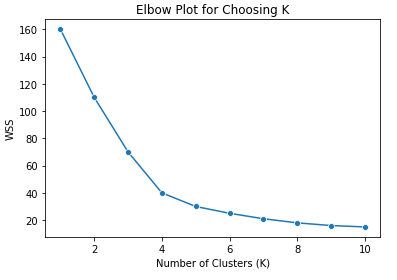# How can you choose the optimal value for ‘k’ in K-Means?

The most common way to choose k is to run the algorithm over a range of values and then plot the within-cluster sum of squares, or a similar evaluation metric, against the values of k. While the within-cluster sum of squares will monotonically decrease as k gets larger, there is usually a point where an elbow-like pattern appears, indicating that increasing k beyond that point produces diminishing returns. This is analogous to overfitting in supervised learning. In the example elbow plot below, k=4 would be the best choice, since the magnitude of decrease in WSS beyond 4 clusters diminishes compared to that up to 4.